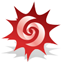# Acoustics and Vibration Animations

Daniel A. Russell, Graduate Program in Acoustics, The Pennsylvania State University

All text and images on this page are ©2013 by Daniel A. Russell and may not used in other web pages or reports without permission.

The content of this page was originally posted on March 18, 2013.The interactive plot on this page is a Mathematica Computable Document Format file and requires the use of the free Wolfram CDF Player.

# Inharmonicity due to Stiffness for Guitar Strings

## Frequencies for a Stiff String

The equation of motion for a stiff string under tension is:

$T\frac{{\partial }^{2}y}{\partial {x}^{2}}-\text{}YS{\kappa }^{2}\frac{{\partial }^{4}y}{\partial {x}^{4}}=\text{}\rho S\frac{{\partial }^{2}y}{\partial {t}^{2}}$

where the first term represents the net force on the string due to the tension T, the second term involves the net force due to the bending moments and shear forces and depends on the elastic modulus (Young's modulus Y), the cross-sectional area S and the radius of gyration κ, and the quantity on the right of the equal sign represents the inertia property (mass per unit length times acceleration) of the string. For a cylindrical string, the radius of gyration equals half the radius, κ = a/2.

At low frequencies (with long wavelengths), the strings behaves as if it were completely flexible, and transverse waves on the string travel with a speed $c=\sqrt{\frac{T}{{\rho }_{\ell }}}$ associated with normal transverse waves on a flexible string. At high frequencies (with short wavelengths) the string acts more like a stiff bar, and transverse waves show a dispersive effect, traveling with the speed of flexural bending waves $v\text{}=\text{}\sqrt{\omega \kappa \sqrt{Y/{\rho }_{\ell }}}$ which depends on frequency.

If the string was perfectly flexible, then the resonances frequencies of the string would be harmonics such that the higher frequencies would be exactly integer multiples of the fundamental frequency, fn = n fo. However, for a stiff string, the frequencies are not exactly integer multiples, and the string exhibits inharmonicity.

Fletcher and Rossing define an inharmonicity constant

$B=\frac{{\pi }^{2}YS{\kappa }^{2}}{T{L}^{2}}=\frac{{\pi }^{2}Y\left(\pi {a}^{2}\right)\left({a}^{2}/4\right)}{T{L}^{2}}$

to demonstrate the effect that bending stiffness has on the frequencies for the strings of a musical instrument. For a string which is "fixed" a both ends with a pinned boundary condition (also called "simply supported), the frequency of the n-th partial of the string is

${f}_{n}=n{f}_{o}\sqrt{1+B{n}^{2}}$

where fo is the fundamental frequency of the string. If the string has clamped boundary conditions at both ends, then the n-th partial of the string is given by

${f}_{n}=n{f}_{o}\sqrt{1+B{n}^{2}}\left(1\text{}+\text{}\frac{2}{\pi }\sqrt{B}+\frac{4}{\pi }B\right)$

In both cases, the frequencies of the string are no longer harmonics.

## Effect of Stiffness on the Inharmonicity of Guitar Strings

The plot below shows the frequencies of a string; if the string behaves as a flexible string, frequencies will be harmonically related and the plot will be a straight line, indicating that fn = n fo. If the string acts as a stiff spring, the plot will curve upward with higher frequencies diverging considerably from the straight line.

The blue curve represents the frequencies for a stiff string pinned at both ends (the green curve is for a stiff string clamped at both ends), and the black dashed curve shows the harmonic relationship for a flexible string using the equations given above.

The inharmonicity constant depends on the length and radius of the string. Moving the sliders in the plot changes the length and the radius of the string, and the shape of the plot changes to reflect the influence of the inharmonicity constant. Below the plot is a crude representation of a guitar, with a red dot to indicate the approximate fret position and string for a guitar, corresponding to the slider locations.

For a long, thin string (like the E4 string fretted at the nut on the headstock) the inharmonicity is negligible, and the frequencies are almost exactly harmonic (at least as far as the stiffness effects are concerned). For a long thick string, (the low E2 string fretted at the nut on the headstock) the inharmonicity is noticeable for the higher harmonics, but not very noticeable for the lowest frequencies. However, for short, thick string (the low E2 string fretted near the sound hole) the stiffness effects are quite large, and the inharmonicity is easily noticeable even for the first few harmonics.

Move the sliders to explore the effect of bending stiffness on the frequencies for guitar strings.

Click on the "+" next to the sliders to see the numerical values and for additional interactive options.

### References

1. Neville H. Fletcher and Thomas D. Rossing, The Physics of Musical Instruments, 2nd Edition, (Springer-Verlag, 1998, reprinted with some corrections, 2010), pg. 64-65.
2. Neville H. Fletcher, "Normal vibration frequencies of a stiff piano string," J. Acoust. Soc. Am., 36(1), 203-209 (1964).